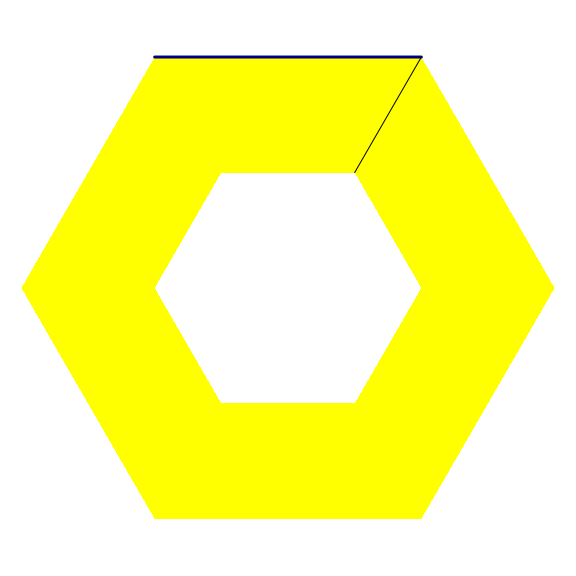# The ‘RCDT’ package.

library(RCDT)

The ‘RCDT’ package is based on the C++ library ‘CDT’. It allows to construct 2D constrained Delaunay triangulations. A constrained Delaunay triangulation is like an ordinary Delaunay triangulation but it can force certain given segments to belong to the triangulation as edges.

For example, it allows to triangulate a concave polygon, by forcing the edges of this polygon to belong to the triangulation. Let’s illustrate this possibility with a pentagram.

# vertices
R <- sqrt((5-sqrt(5))/10)     # outer radius
r <- sqrt((25-11*sqrt(5))/10) # circumradius of the inner pentagon
k <- pi/180 # factor to convert degrees to radians
X <- R * vapply(0L:4L, function(i) cos(k * (90+72*i)), numeric(1L))
Y <- R * vapply(0L:4L, function(i) sin(k * (90+72*i)), numeric(1L))
x <- r * vapply(0L:4L, function(i) cos(k * (126+72*i)), numeric(1L))
y <- r * vapply(0L:4L, function(i) sin(k * (126+72*i)), numeric(1L))
vertices <- rbind(
c(X[1L], Y[1L]),
c(x[1L], y[1L]),
c(X[2L], Y[2L]),
c(x[2L], y[2L]),
c(X[3L], Y[3L]),
c(x[3L], y[3L]),
c(X[4L], Y[4L]),
c(x[4L], y[4L]),
c(X[5L], Y[5L]),
c(x[5L], y[5L])
)
# constraint edges: indices
edges <- cbind(1L:10L, c(2L:10L, 1L))
# constrained Delaunay triangulation
del <- delaunay(vertices, edges)
# plot
opar <- par(mar = c(0, 0, 0, 0))
plotDelaunay(
del, type = "n", asp = 1, fillcolor = "distinct", lwd_borders = 3,
xlab = NA, ylab = NA, axes = FALSE
)par(opar)

Since the polygon is triangulated, it is easy to get its area. It is given by the function delaunayArea:

delaunayArea(del)
#>  0.3102707
sqrt(650 - 290*sqrt(5)) / 4 # exact value
#>  0.3102707

Another possibility offered by the constrained Delaunay triangulation is the triangulation of a “donut polygon”, roughly speaking a “polygon with holes”. Let’s see an example.

nsides <- 6L
angles <- seq(0, 2*pi, length.out = nsides+1L)[-1L]
outer_points <- cbind(cos(angles), sin(angles))
inner_points <- outer_points / 2
points <- rbind(outer_points, inner_points)
# constraint edges
indices <- 1L:nsides
edges_outer <- cbind(
indices, c(indices[-1L], indices[1L])
)
edges_inner <- edges_outer + nsides
edges <- rbind(edges_outer, edges_inner)
# constrained Delaunay triangulation
del <- delaunay(points, edges)
# plot
opar <- par(mar = c(0, 0, 0, 0))
plotDelaunay(
del, type = "n", asp = 1, axes = FALSE, xlab = NA, ylab = NA,
fillcolor = "yellow", lwd_borders = 3, col_borders = "navy"
)par(opar)

The area of the outer hexagon is 3*sqrt(3)/2 and the area of the inner hexagon (the hole) is 3*sqrt(3)/8. Let’s check:

delaunayArea(del)
#>  1.948557
3*sqrt(3)/2 - 3*sqrt(3)/8
#>  1.948557